Function Repository Resource:

# BidiagonalDecomposition

Compute the bidiagonal decomposition of a numerical matrix

Contributed by: Jan Mangaldan
 ResourceFunction["BidiagonalDecomposition"][m] gives the bidiagonal decomposition for a numerical matrix m as a list of matrices {q,b,p}, where q and p are orthonormal matrices and b is an upper bidiagonal matrix.

## Details

The bidiagonal decomposition of a matrix is often used as the first step in computing the singular value decomposition.
The matrix m may be rectangular.
The original matrix m is equal to q.b.ConjugateTranspose[p].
q and p are column orthonormal matrices, whose transposes can be considered as lists of orthonormal vectors.

## Examples

### Basic Examples (1)

Compute the bidiagonal decomposition for a 3×2 numerical matrix:

 In:=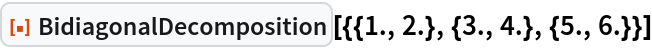Out=In:=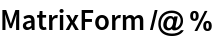Out=### Scope (2)

m is a 3×4 matrix:

 In:=Find the bidiagonal decomposition of m using machine-number arithmetic:

 In:=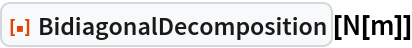Out=In:=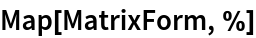Out=Find the bidiagonal decomposition of m using 24-digit precision arithmetic:

 In:=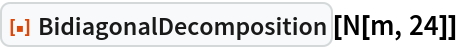Out=The bidiagonal decomposition of a random complex-valued 2×4 matrix:

 In:=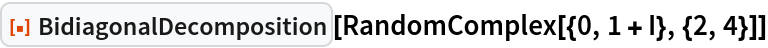Out=### Properties and Relations (5)

m is a random matrix with 3 columns:

 In:=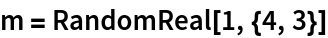Out=Find the bidiagonal decomposition of m:

 In:=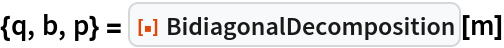Out=Verify that m is equal to q.b.ConjugateTranspose[p]:

 In:=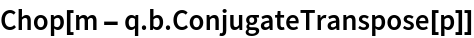Out=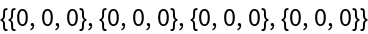Verify that q and p are unitary:

 In:=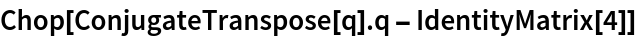Out=In:=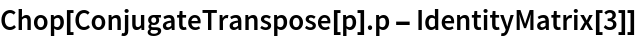Out=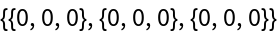b has the same singular values as m:

 In:=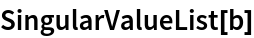Out=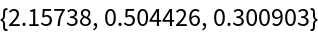In:=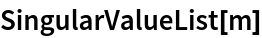Out=## Version History

• 1.0.0 – 24 January 2022